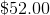# ISEE Middle Level Math : How to find percentage

## Example Questions

### Example Question #151 : Percentage

Convert the fraction to a percentage.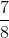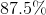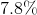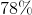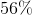Explanation:

To convert a fraction to a percentage, divide the numerator of the fraction by the denominator.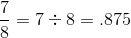Move the decimal in the quotient two places to the right to create a percentage.### Example Question #152 : Percentage

What percentage does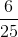represent?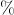Explanation:

To get a fraction into a percentage you must turn it into a decimal.

Make the fraction overso you must multiple it by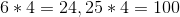.

This gives you the decimal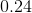which you multiply byto give you.

### Example Question #153 : Percentage

What is 0.036 as a percent?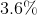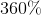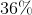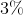Explanation:

Method 1:

Rewrite 0.036 as a fraction: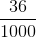Because percents are based on 100, divide the numerator and denominator by 10.

3.6%

Method 2:

Multiply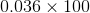To multiply by 100, move the decimal point two places to the right; then add the percent sign:

### Example Question #154 : Percentage

75 out of 500 is what percent?Explanation:represents part of the whole which is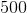.  The fraction would be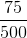Then, set up a proportion: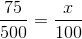represents the percent

Cross multiply: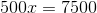Divide both sides by the coefficient of x, which is 500: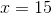### Example Question #155 : Percentage

What is 0.467 as a percent?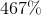There is no solution.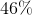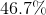Explanation:

Method 1:

Rewrite 0.467 as a fraction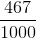Divide numerator and denominator by 10.

46.7 %

Method 2:

Multiply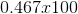Move the decimal in 0.467 to the right and add a % sign.

### Example Question #156 : Percentage

What isof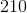?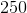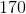Explanation:

To solve, multiply: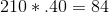Answer:### Example Question #157 : Percentage

What is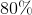of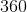?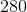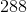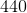Explanation:

To solve, multiply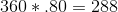Answer:### Example Question #158 : Percentage

What is the percentage equivalent of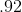?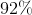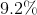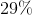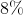Explanation:

To solve, multiply: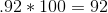Answer:### Example Question #159 : Percentage

What is the percentage equivalent of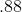?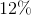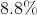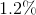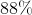Explanation:

To solve, multiply: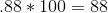Answer:### Example Question #160 : Percentage

A bicycle is on sale for 35% off the original price of \$80.00. What is the sale price of the bicycle?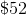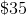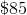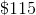Explanation:

To solve, first multiply: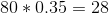Then, subtract that amount from the original price: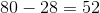Answer: The sale price is